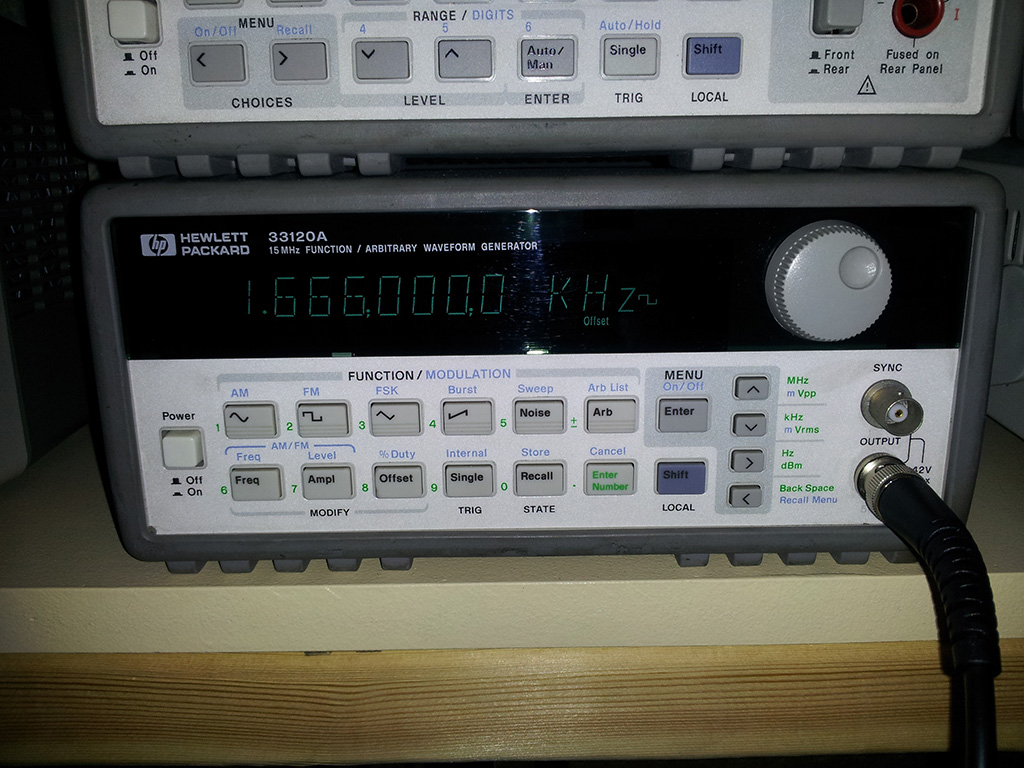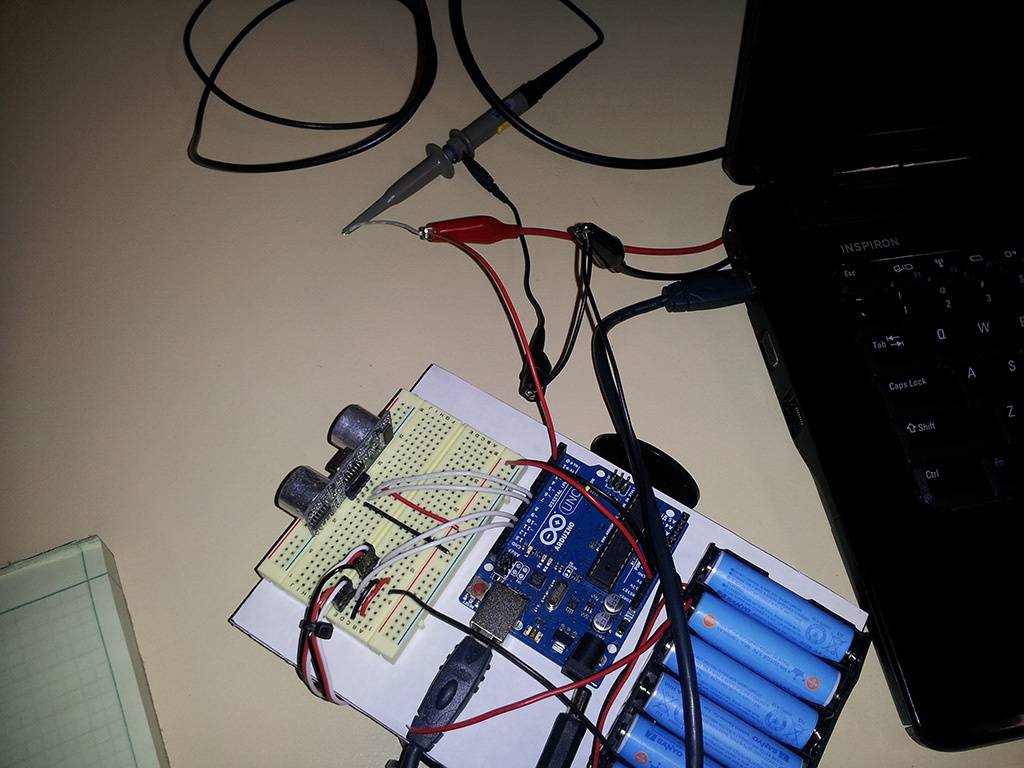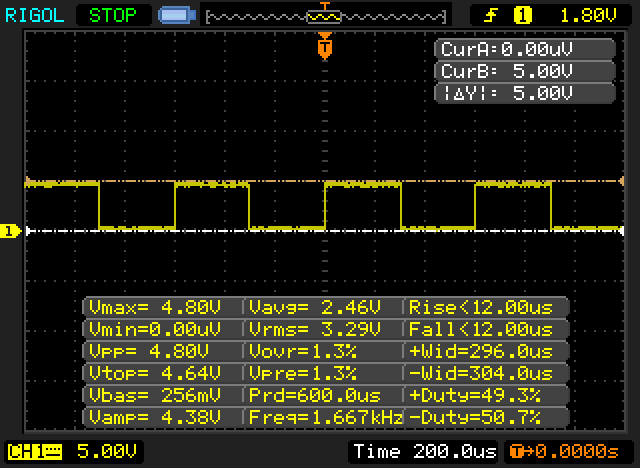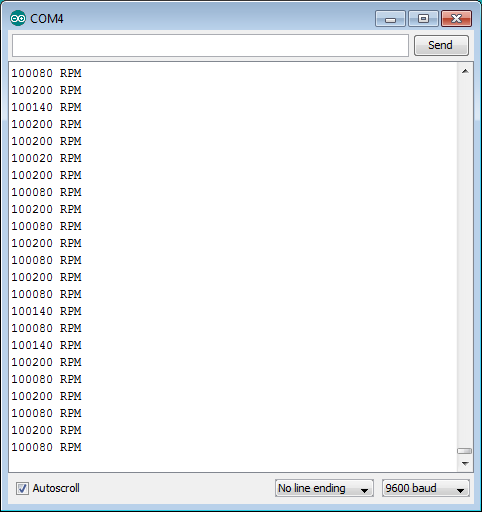# Measuring RPMs with Arduino

Posted by Nathan House, in Programming, Electronics 12 June 2013 · 13,256 views

I was asked whether an Arduino would be capable of measuring a device that is rotating at 100,000 RPM. Assuming that there is 1 interrupt per rotation, that gives us the following:

Therefore,

So the period is and the frequency =

I set my function generator to a 1666Hz square wave:Then connected the output to my Arduino and oscilloscope:The oscilloscope shows the signal:It's pretty close to what we want, although it looks like the frequency is a tad high (I'm betting on the function generator being off, not the scope, since it's fairly old).

So what we want is to do now is use a hardware interrupt on the Arduino to record the number of pulses per second (which we'll multiply by 60 to get the number of pulses per minute).

Here's the code to do that:
const unsigned long serialPeriod = 1000;       // print to the serial console every second
unsigned long timeSerialDelay = 0;

volatile unsigned long numInterrupts = 0;

void setup()
{
Serial.begin(9600);

attachInterrupt(0, isr, FALLING); // interrupt on falling edge
}

void loop()
{
outputRPM();
}

void isr()
{
numInterrupts++;
}

void outputRPM()
{
if((millis() - timeSerialDelay) >= serialPeriod)
{
unsigned long RPM = (numInterrupts*60);

Serial.print(RPM);
Serial.println(" RPM");

numInterrupts = 0;
timeSerialDelay = millis();
}
}


Uploading the code to the Arduino and opening the serial monitor results in this being shown:The RPM measurements range from 100,080 RPM to 100,200 RPM, which is a little high.

The error is

Remember that the oscilloscope said the frequency was a little high, though, so the measurement may actually be correcter (yeah, I just made up that word).

Conclusion: it seems possible to measure interrupts at 1.7kHz pretty accurately with an Arduino. More testing would need to be done (with calibrated equipment) to determine how accurate the measurements can be, and I'm also sure that the Arduino code I posted could be optimized.

• 0

### Categories

•Uncategorized (9)
•Automotive (1)
•Balancing Robot #2 (2)
•Books (1)
•CNC (3)
•Completed Projects (7)
•Electronics (7)
•Programming (3)
•Site News (1)

### Tags